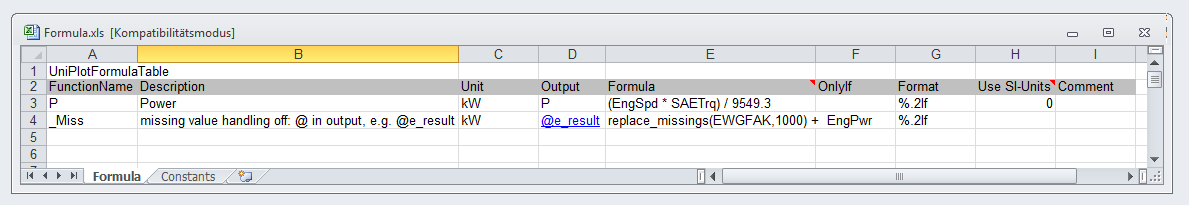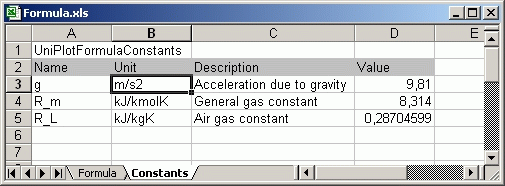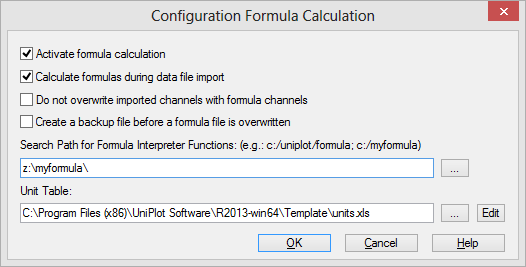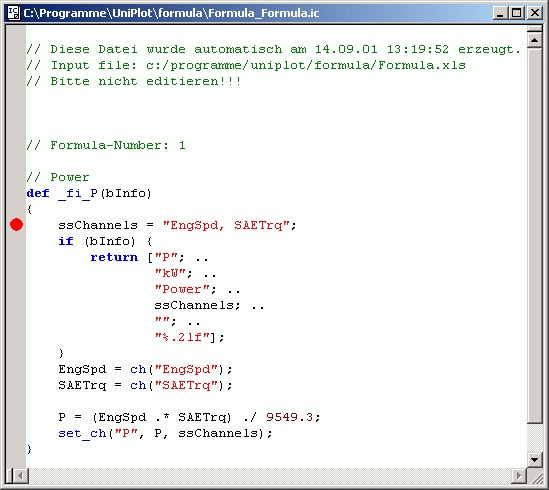# Formula Interpreter 2 - Creating a formula table¶

Chapter Formula Interpreter 1 - Introduction contained a brief description of the formula table. In this chapter we would like to describe it in more detail.

## The Formula Table¶

Each row in the table contains a formula. The table is especially suitable for simple and short formulas. Excel can be used to create and edit the table. The following figure shows a sheet with one formula:Cell A1 must contain the string `UniPlotFormulaTable` or `UniPlotFormelTabelle`.

The second row contains the column headers.

The table can contain empty and comment rows. A comment row must start in column A with a # character. This can be used to disable formulas.

An example Excel file can be found in the directory `<uniplot>\formula`.

The table contains 9 columns:

### 1. Column - FunctionName¶

The function name of the formula. It can be differently from the channel name. The name must begin with an alphabetic character, an underscore (`_`), followed by zero or more alphanumeric characters including the underscore. Case is significant. The name should be unique. It should not appear again in the first column. It should not have also been used in any other ic file.

### 2. Column - Description¶

A brief formula description. It will be saved in the channel attribute `Description` in the NC file.

### 3. Column - Unit¶

A string that specifies the units used for the channel’s data. The units will be saved in the channel attribute `units` in the NC file.

### 4. Column -Output¶

This column contains the name of the result channel. The name is used to create a variable in the NC file. If the name already exists in the file, the channel data will be overwritten.

The name must begin with an alphabetic character or an underscore, followed by zero or more alphanumeric characters including the underscore (<tt>_</tt>) or numbers. Case is significant.

Option: Missing Value Handling

To disable the missing value handling add a `@` character to the output name. for example `@P_kor`. The missing value handling must be disabled if you want to replace missing values by invoking the replace_missing() function.

### 5. Column - Formula¶

This column contains the formula definition. Constant values in formulas must contain a period (.) as a decimal separator.

### 6. Column - OnlyIf¶

In this column a condition can be indicated which will be used to decide whether the formula is to be applied to the active NC-file. If no condition is indicated (empty field), the frame work will try to evaluate the formula. How conditions are defined is described in the chapter Definition of a Condition. Examples of typical conditions:

`diesel`: Calculate this channel only with diesel engines.

`otto`: Calculate this channel only with petrol engines.

`turbodiesel`: Calculate this channel only with turbo charged diesel engines.

### 7. Column - Numberformat¶

Format specification, e.g `%e` or `%.4f`. Further information about the format can be found in the documentation of printf. The format string is saved in channel attribute `C_format` in the NC file.

### 8. Use SI-Units¶

This column must contain the value 1 if the formula should be calculated using SI units. If set to 1, the channels used in the formula will be converted to SI units before the formula is evaluated. The formual result will be converted to the unit specified in column 3. If one of the channel units is not found in the unit table, the formula will not be evaluated. See Unit Converter.(New in UniPlot R2013.11.)

### 9. Column - Comment¶

This column can contain a comment. Before R2013.11 the comment was in column 8.

## The Constant Table¶

This table contains constants. Excel has been used to specify the constants, as shown in the following figure.The constant names have to be unique. They should not be identical to any channel or attribute name used in your data files.

Before the function ch retrieves data for a given name it checks if a channel with this name already exists in the specified NC file. If a channel is not found the ch function tries to read the data from the global attributes in the NC file (header data). If this fails because a global attribute does not exist, the function checks for a constant with the given name in the constant table and returns its value.

The cell “A1” contains the string `UniPlotFormulaConstants` or `UniPlotFormelKonstanten`. The second row contains column titles.

The following rows contain constants. Each row contains 4 columns:

1. Column - Name
Constant name
2. Column - Unit
Constant unit.
3. Column - Description
Brief description
4. Column - Value
Constant value.

The table can contain empty and comment rows. A comment row must start in column A with a `#` character. This can be used to disable formulas.

The constant table can be saved as a separate document or as a sheet in the formula document. The constant table will be converted as well during the formula conversion. To speed up access, the constant values will be saved in a UniPlot map.

## Formula Configuration¶

• Start UniPlot.

• Choose Tools=>Formula Configuration.• Specify the search path where your formula files are located.

• Press OK.

If the table contains invalid formulas, a log file with error and warning messages is created. If all formulas are converted they can be applied to the data files.

Formulas are saved as UniScript functions. The file name is made up of the Excel file name plus sheet name and the extension `.ic`. Do not edit the file because it will be overwritten without notice the next time you convert the Excel formula file.

## Debug Formula¶

To debug a formula, open the appropriate IC file. To set a breakpoint, click on a row inside the function and press F9. If a breakpoint cannot be set, click F4 to translate the function and then press F9 again. A red dot will be displayed at the left border.

Open the data browser and press F9 to update the formulas. If the function can be calculated (all dependent channels are available in the file) the execution will stop at the breakpoint.Use the F10 key to can step through instructions and the F11 key to enter into a function. To display a variable value, place the mouse cursor over a variable name.

For additional information, see The Debugger.

To next chapter Formula Interpreter 3 - Creating Script Formulas.

id-6196